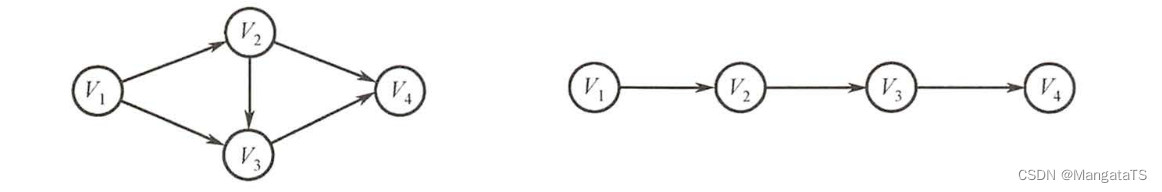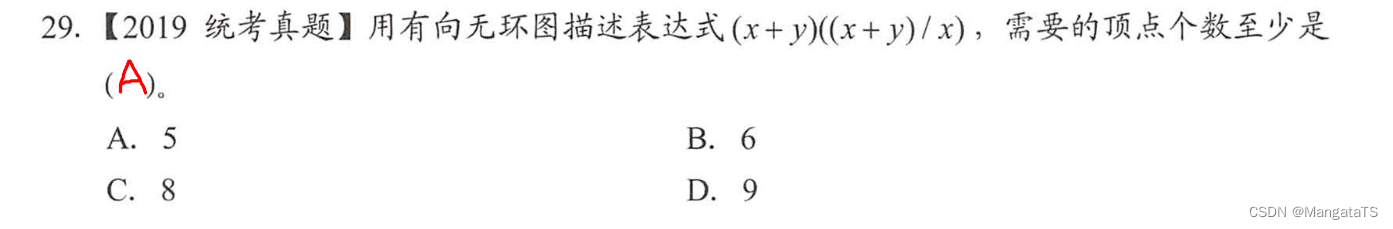# 考研数据结构与算法（七）图论¶

## 一、图的基本概念¶

### 1.2 基本术语¶

#### 1.2.5 完全图¶

• 在一个顶点数量为 $$n$$ 的无向图中，若边的数量为 $$\frac{(n-1)\times n}{2}$$ ，则该无向图为完全图
• 在一个顶点数量为 $$n$$ 的有向图中，若边的数量为 $$(n-1)\times n$$ ，则该有向图为完全图

#### 1.2.7 连通、连通分量、连通图¶

• 连通：在无向图中若从顶点 $$u$$ 到顶点 $$v$$ 有路径存在，那么称 $$u$$$$v$$ 是连通的
• 连通图：若无向图中任意两点是连通的，那么这个无向图就称为连通图，否则称为非连通图
• 连通分量：对于一个连通图而言，其连通分量只有一个就是其本身，而对于非连通图而言，连通分量有多个，其每一个子图（或者称为极大联通子图）都是一个连通分量

#### 1.2.8 强连通¶

• 强连通：在有向图中，若从顶点 $$v$$ 到顶点 $$w$$ 和从顶点 $$w$$ 到顶点 $$v$$ 之间都有路径 ，则称这两个顶点为强连通
• 强连通图：若有向图中任意两点之间都是强连通的，那么称这个有向图为强连通图，否则称为非强连通图
• 强连通分量：对于一个强连通图而言，其强连通分量就是本身，而对于一个非强连通图而言，其极大强连通子图就为该非强连通分量（在子图中任意两点仍满足强连通

#### 1.2.10 顶点的度¶

• 无向图：对于无向图而言，顶点的度就是和该顶点相连接的边数
• 有向图：对于有向图而言，指向该顶点的边数称为入度，而从该顶点指出的边数称为出度
• 无向图的全部顶点的度之和等于两倍的边数
• 有向图的全部顶点的出度等于全部顶点的入度等于边数

#### 1.2.12 稠密、稀疏图¶

• 稠密图：点数较大，而边数较少的图称为稀疏图
• 稠密图：点数较小，而边数较多的图称为稠密图

#### 1.2.13 路径、路径长度、回路¶

• 路径：顶点 $$V_u$$ 到顶点 $$V_v$$ 的顶点序列 $$V_p,V_{i1},V_{i_2},……,V_u$$ 称为这两点的路径
• 路径长度：路径上边的数目称为路径长度
• 回路：起点和终点相同的非 $$0$$ 路径长度的路径称为回路

#### 1.2.14 简单路径、简单回路¶

• 简单路径：在路径的顶点序列中顶点不重复的路径称为简单路径
• 简单回路：除了起点和终点相同外其余顶点不重复的回路称为简单回路

## 二、图的存储结构¶

### 2.1 邻接矩阵¶

$A[i][j] = \begin{cases} 1 ,若(V_i,V_j)或者 <V_i,V_j>是E(G)中的边 \\ 0 ,若(V_i,V_j)或者 <V_i,V_j>不是E(G)中的边\\ \end{cases}$

$A[i][j] = \begin{cases} V[i][j] ,若(V_i,V_j)或者 <V_i,V_j>是E(G)中的边 \\ 0或∞ ,若(V_i,V_j)或者 <V_i,V_j>不是E(G)中的边\\ \end{cases}$

#define MaxVertexNum 100
typedef struct {
int Vex[MaxVertexNum];//存储当前顶点的表
int Edge[MaxVertexNum][MaxVertexNum];//邻接矩阵表
int vexnum, arcnum;//当前的顶点数和弧数
}


### 2.2 邻接表¶

#define MaxVertexNum 100
typedef struct ArcNode{ //边表结点
struct ArcNode *next; //下一条弧的位置
// infoType info;
}ArcNode;
typedef struct VNode{ //顶点结点
VertexType data; //顶点的信息，例如值之类的
ArcNode *first;//第一个边表结点的位置
typedef struct{
int vexnum,arcnum;//顶点数和弧数
} ALGraph;//封装的邻接表的图


### 2.3 十字链表¶

• 顶点结点有三个域

• data 域存放顶点相关的数据信息，
• firstin 域指向以该顶点为弧头的第一个弧结点（比如这个结点是第二个结点，那么就指向第二列，从上往下看的第一个弧节点元素，当然若是没有的话则指向NULL
• firstout 域指向以该顶点为弧尾的第一个弧结点（比如这个结点是第三个结点，那么就指向第三行 的第一个弧结点元素）
• 弧结点有五个域

• tailvex 尾域表示弧尾的位置（显然对于某一行数据来说，弧尾域都是一样的）
• headvex 头域表示弧头的位置（显然对于某一列数据来说，弧头域都是一样的）
• hlink 链域指向弧头相同的下一条弧（其实就是对于每一列的弧结点元素来说，上下相邻的元素从上往下指）
• tlink 链域指向弧尾相同的下一条弧（其实就是对于每一行的弧结点元素来说，左右相邻的元素从左往右指）
• info 域一般用于弧的值的存储

### 2.4 邻接多重链表¶

• 顶点结点有两个域

• data
• firstedge 用于存储与该结点相连的第一条边
• 边结点有六个域（但是一般只用其中间的四个）

• mark 域用于标记这个边是否进行操作过，$$0$$ 表示没操作过，$$1$$ 表示操作过了
• ivex 用于表示该边的其中一个结点在图中的下标
• ilink 用于表示与结点 ivex 相邻的第一条边
• jvex 用于表示该边的另一个结点在图中的下标
• jlink 用于表示与结点 jvex 相邻的第一条边
• info 用于表示该边的一些权值等信息

## 三、图的遍历方法¶

### 3.1 广度优先搜索（BFS）¶

bool vis[N];
void bfs(int s){
queue<int> que;
que.push(s);
vis[s] = true;
while(!que.empty()){
int t = que.front();
que.pop();
printf("%d\t",t);
for(int i = 0,l = V[t].size();i < l; ++i) {
if(!vis[V[t][i]]){
que.push(V[t][i]);
vis[V[t][i]] = true;
}
}
}
}

void BFS_Traverse(int n) {
memset(vis,false,sizeof vis);
for(int i = 1;i <= n; ++i) {
if(!vis[i]) bfs(i);
}
}


int dis[N];
bool vis[N];
int bfs(){
memset(dis,0x3f,sizeof dis);
queue<int> que;
dis = 0;
que.push(1);
vis = true;
while(!que.empty()){
int t = que.front();
que.pop();
if(t == n)
return dis[n];
for(int i = 0,l = V[t].size();i < l; ++i) {
if(!vis[V[t][i]]){
que.push(V[t][i]);
dis[V[t][i]] = dis[t] + 1;
vis[V[t][i]] = true;
}
}
}
return -1;
}


ps ： ① 如果是想输出这个最短路径的话，我们可以用一个数组记录每一步的前一个点的位置，然后递归输出一下即可 ② 要是想获取层次遍历的结果，只需要在队首元素每次取出的时候输出即可

### 3.2 深度优先搜索（DFS）¶

$$DFS$$ 即优先深度搜索，可能会从一个点开始往一个方向一直往下搜，然后搜到尽头后就回溯，直到当前连通块的所有的结点全都被搜索过，就停止递归搜索，一般来说 $$DFS$$ 的代码都会十分简洁，但是常数耗时会非常大

bool vis[N];//结点被访问情况
vector<int> Edge[N];//存储的边
void DFS(int s){//当前访问的结点
if(vis[s]) return;
vis[s] = true;//标记访问
//do something
for(int i = 0,l = Edge[s].size();i < l; ++i) {
int v = Edge[s][i];
dfs(v);//递归搜索
}
}

void DFS_Traverse(int n) {
memset(vis,false,sizeof vis);
for(int i = 1;i <= n; ++i) {
if(!vis[i]) dfs(i);
}
}


• 邻接矩阵存储：时间复杂度为 $$O(|V|^2) = O(n^2)$$ ，空间复杂度为 $$O(|V|) = O(n)$$
• 邻接表存储：时间复杂度为 $$O(|V| + |E|) = O(n + e)$$ ，空间复杂度为 $$O(|V|) = O(n)$$

## 四、图的基本应用¶

### 4.2 最短路径¶

https://acmer.blog.csdn.net/article/details/123040285

### 4.3 有向无环图描述表达式¶

$( ( a + b) * ( b * ( c + d)) + ( c + d) * e) * ( ( c + d) * e)$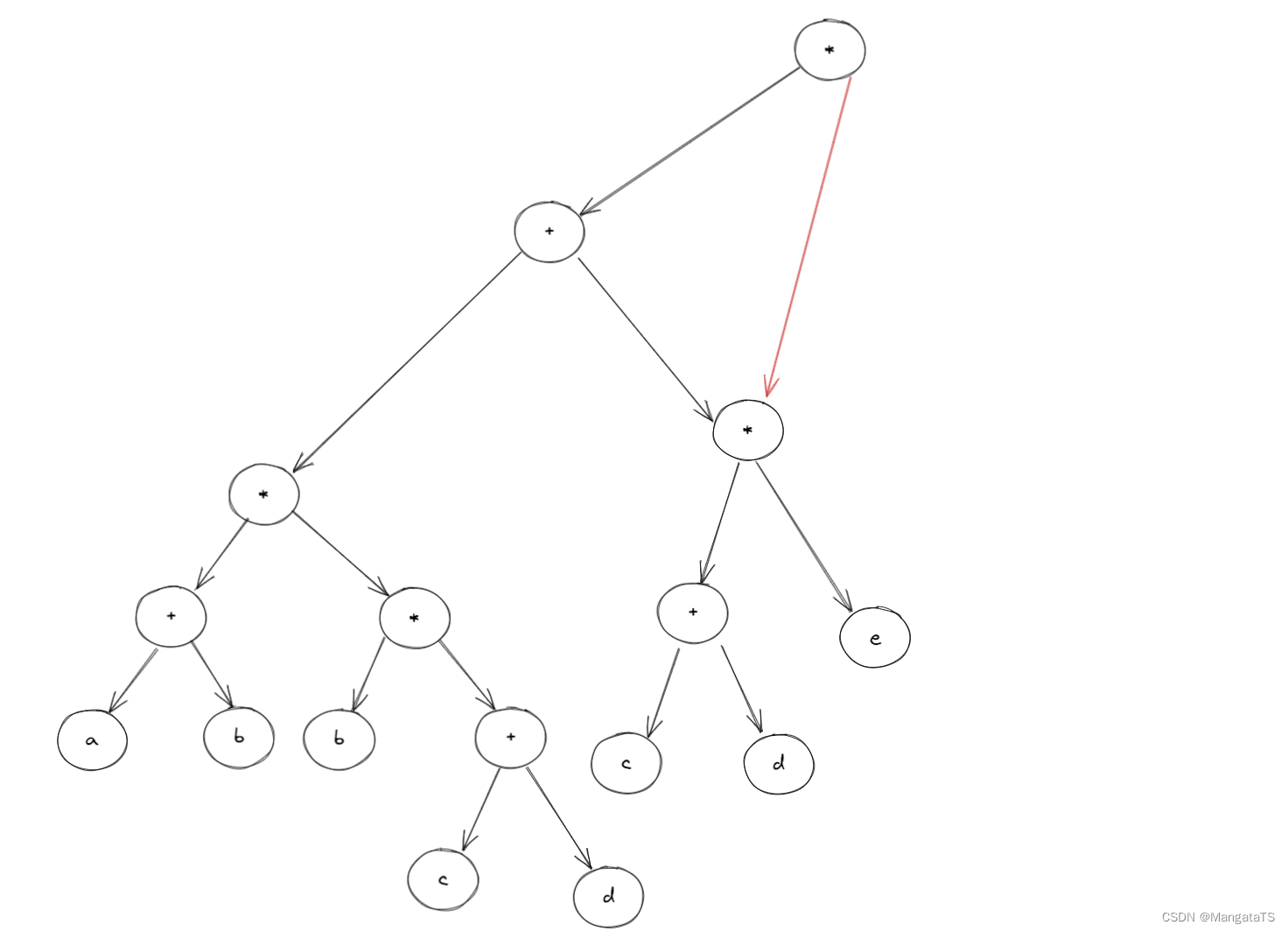然后我们发现子树结构 $$(c+d)$$ 其实也是重复的，于是我们决定删除一个，就得到了如下结构：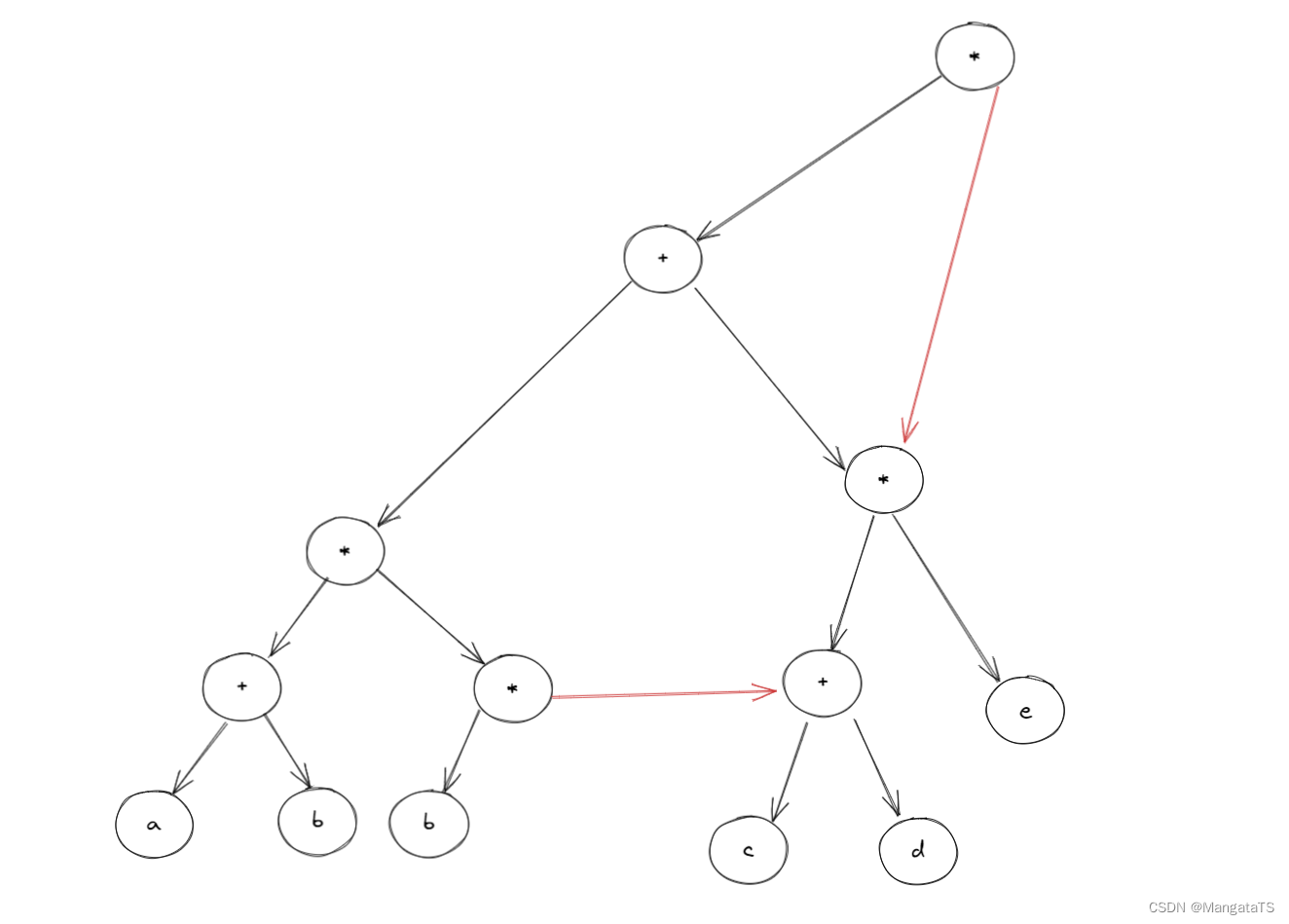然后我们发现结点 $$b$$ 其实也是重复的，于是我们删除一个就得到了：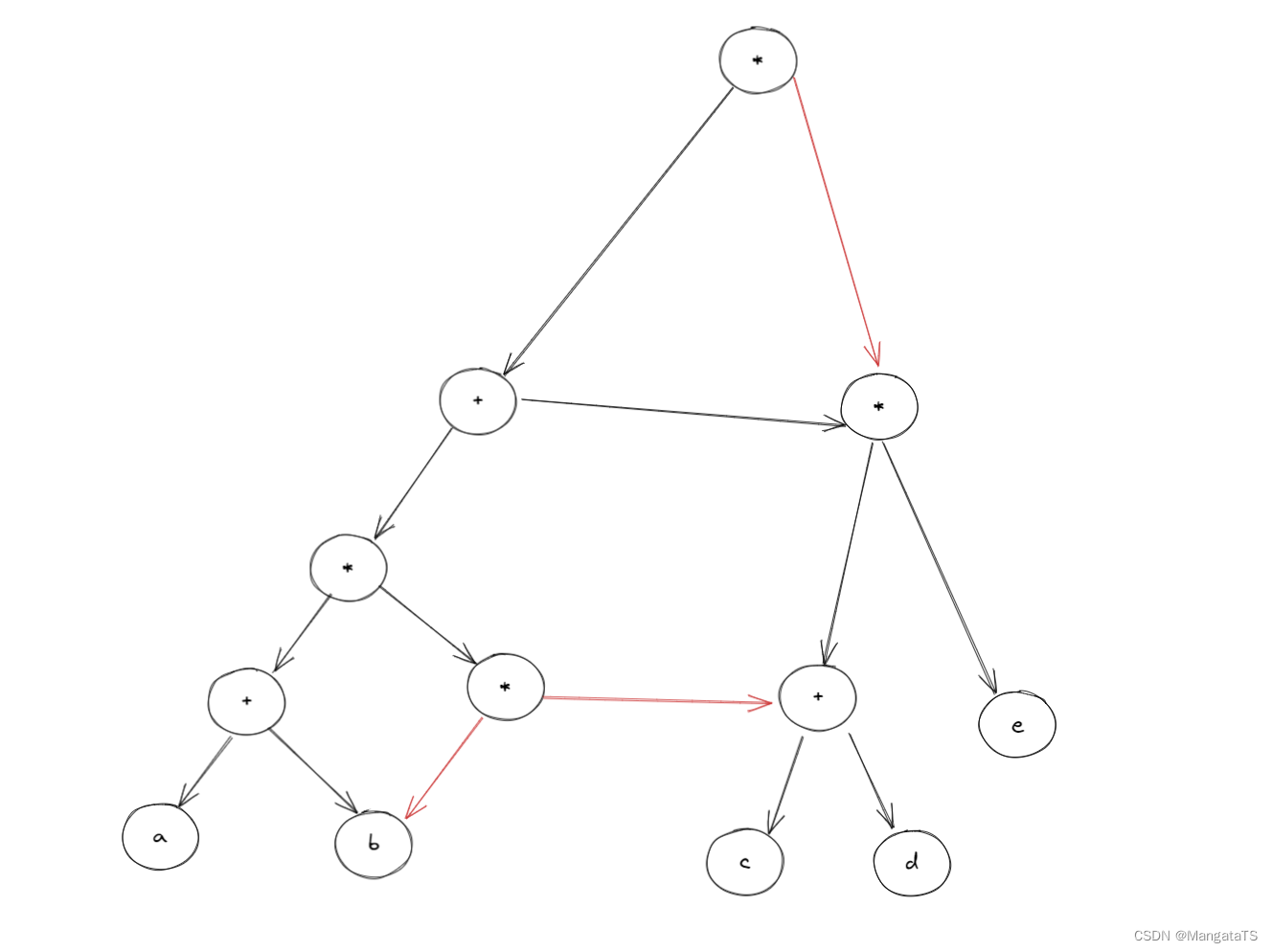我们发现化二叉树为 $$DAG$$ 图的时候实际上就是将二叉树重复的子树删掉，并且将分支结点指向其中一个子树即可

## 五、错题¶

### 选择题¶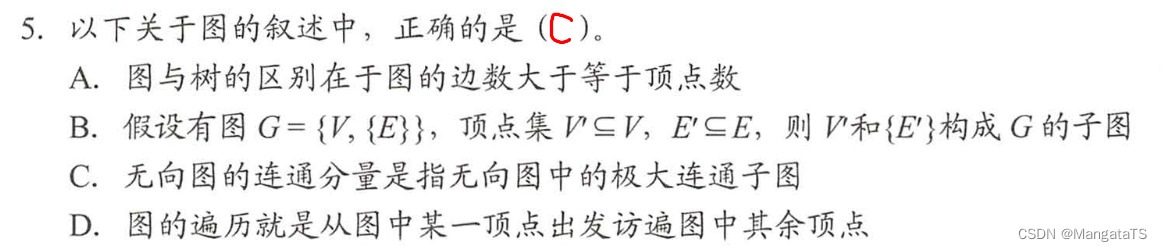$$E'$$ 中的边对应的顶点不是 $$V'$$ 的元素， $$V'$$$${E'}$$ 无法构成图，故选项 $$B$$ 错 且若图非连通， 则从某一顶点出发无法出问到其他全部顶点，选项 $$D$$ 的说法不准确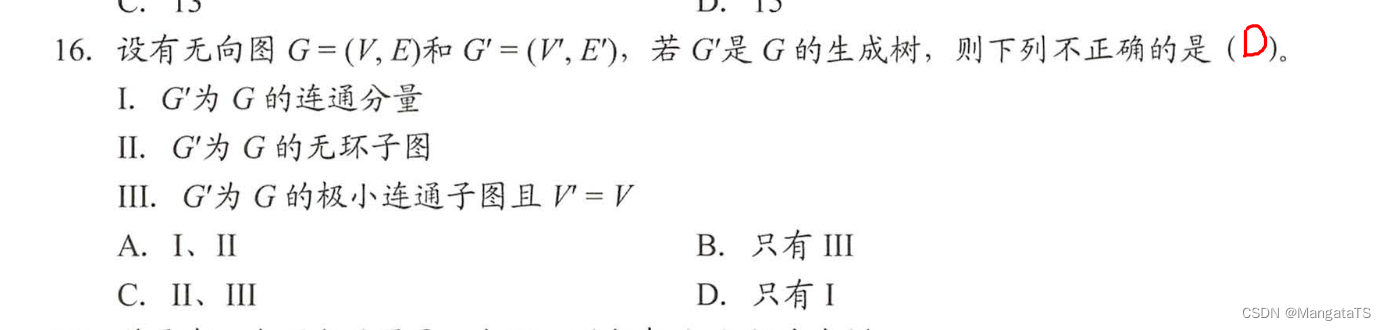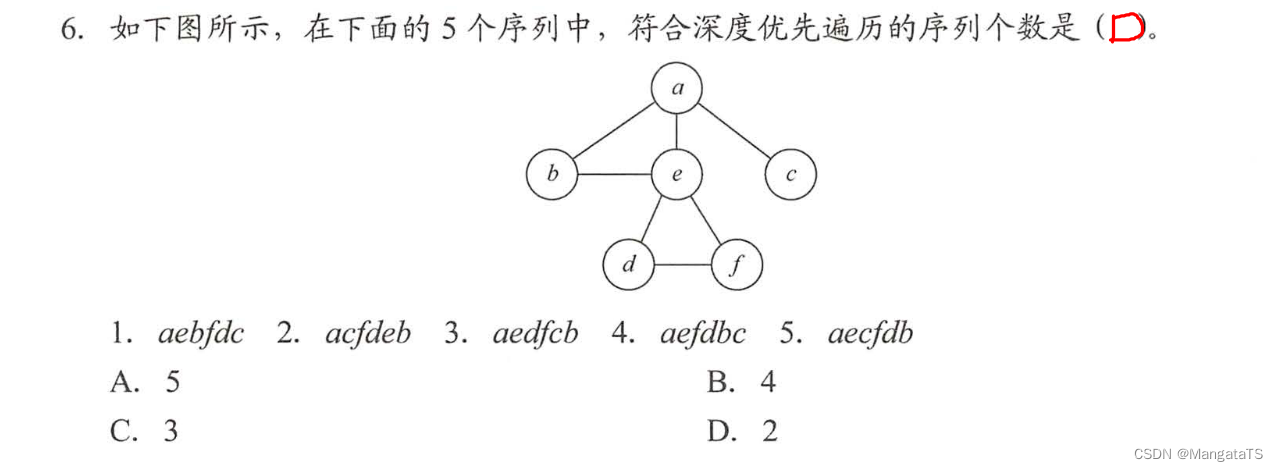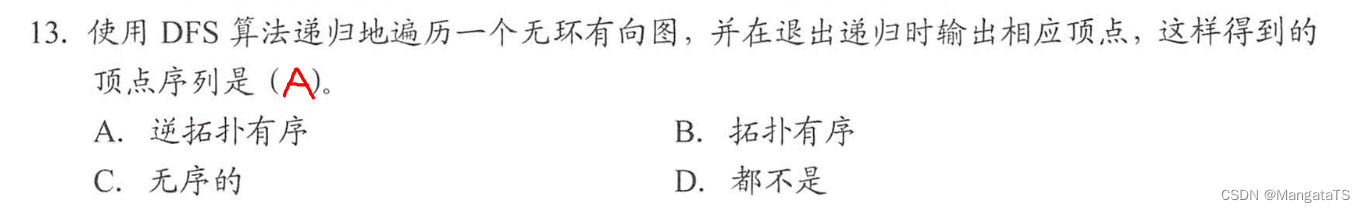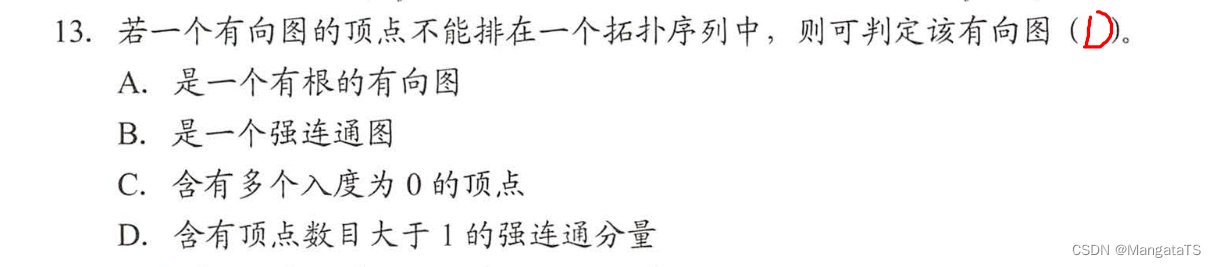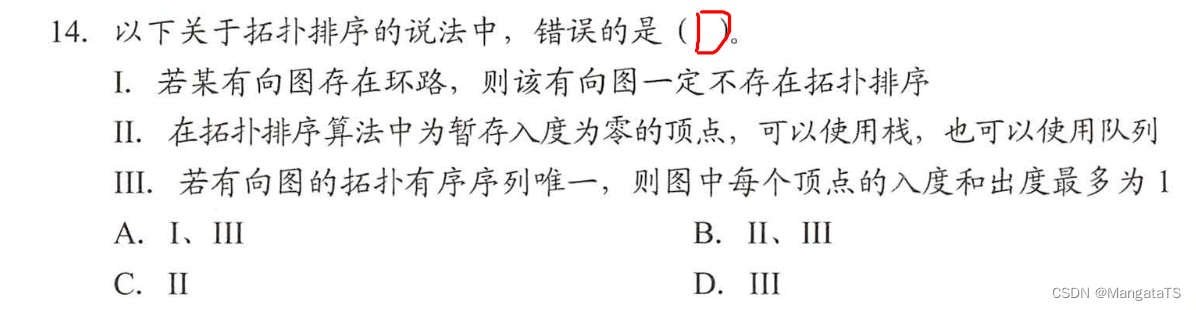• $$Ⅰ$$ 中，对于一个存在环路的有向图，使用拓扑排序算法运行后，肯定会出现有环的子图，在此环中无法再找到入度为 $$0$$ 的结点，拓扑排序也就进行不下去。
• $$Ⅱ$$ 中，注意，若两个结点之间不存在祖先或子孙关系，则它们在拓扑序列中的关系是任意的（即前后关系任意），因此使用栈和队列都可以，因为进栈或队列的都是入度为 $$0$$ 的结点，此时入度为 $$0$$ 的所有结点是没有关系的。
• $$Ⅲ$$ 中，若拓扑有序序列唯一，则很自然地让人联系到一个线性的有向图（错误），下图的拓扑序列也是唯一的，但度却不满足条件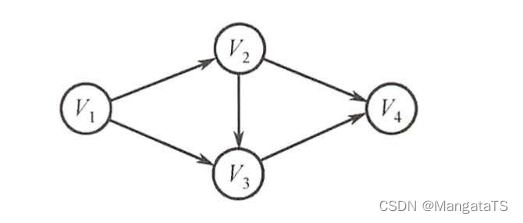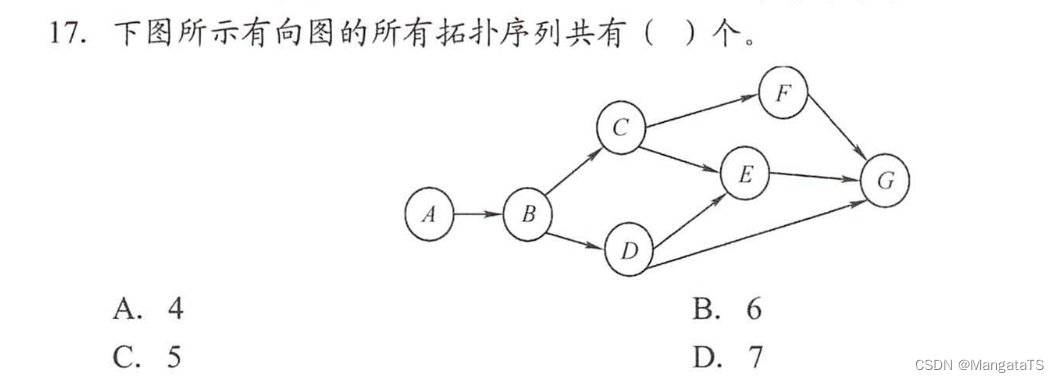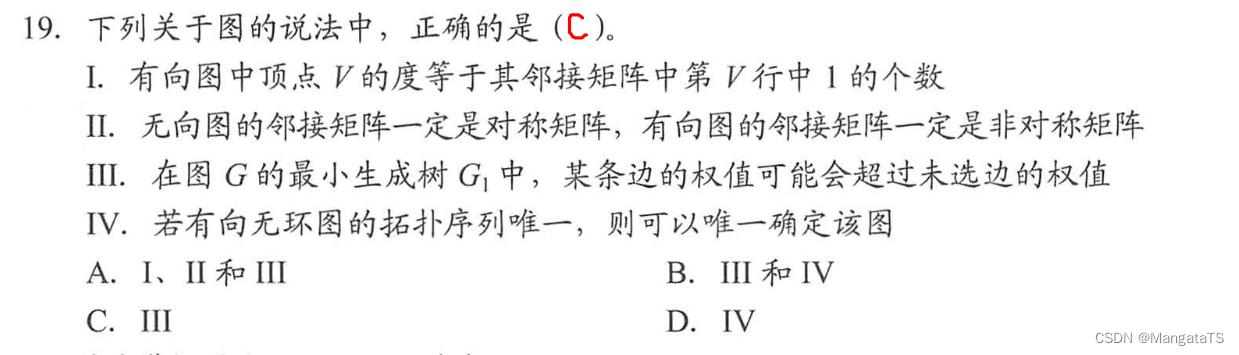• $$Ⅰ$$ ：有向图中的度为入度加出度，而矩阵中第 $$V$$ 行只能代表点 $$V$$ 的出度数
• $$Ⅱ$$ ：无向图的邻接矩阵一定是对称矩阵，有向图的邻接矩阵也有可能是对称矩阵
• $$Ⅲ$$ ：最小生成树中的 $$n-1$$ 条边并不能保证是图中权值最小的 $$n - 1$$ 条边， 因为权值最小的 $$n-1$$ 条边并不一定能使图连通，所以会存在某条边权值超过未选的边
• $$Ⅳ$$ ：有向无环图的拓扑序列唯一并不能唯一确定该图。在下图所示的两个有向无环图中，拓扑序列都为 $$V_1, V_2, V_3,V_4$$